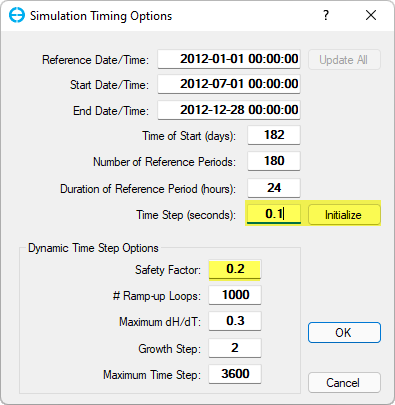# How to calculate CFL Time Step

Hi I’m user of graduated student.
I have some question for this model,

I want to know how to calculate the Time Step in EFDC source code.
I wonder that CFL number is calculated in this process such as
(\delta x * u)/\delta t + (\delta y * u)/\delta t + (\delta z * u)/\delta t < 1
but I calculated it with hand, it is quite different with EFDC time step CFL number.

i have a very simple gird that x, y, z is 100m20m1m
and grid size is 2m1m (grid number 5020)
and when i calculated it with my hand to use that equation, i got 1500s
but in the efdc model, that suggest 0.14s CFL number.
how i supposed to know that time step in EFDC?

thanks.

Hi hgks,
For the models using dynamic time step option, The EFDC code actually calculates the time step by combining several methods:

• Method 1: based on Courant–Friedrichs–Lewy condition
• Method 2: based on Positivity of advected material (when the model includes one of temperature, salinity, dye, etc. module)
• Method 3: based on Limit rate of depth change
The final time step is chosen as the minimum of the three method for all the grid cells, then multiplied by the Safety Factor (see the attached photo).
It is noted the initial time step (highlighted in yellows) is usually set by a small value (smaller than the one generated by EE). This initial time step will be updated over time during the simulation based on the EFDC’s estimation described above.Sincerely,
Kien

hi, thank you for your comment for me.
I have one more question for time step setting,

1. Can I set a time step interval arbitrarily?
2. If you look at the last 3rd method you told me, it is defined as the value of TREF/NTSPTC, so can I change the value of TREF or NTSPTC in the source code card images 7 and 8?
3. Is it possible to change the TREF value because it is fixed to 86400(s)?
4. Even though the grid is large and not deep, the time interval provided by EE was presented too small (I can’t set it for more than half a second), so I wanted to increase this time interval, but in this case, how should I solve it?
5. What is the relationship between safe factor and time step?
Can I increase the time step value by setting the safe factor high?